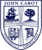JOHN CABOT UNIVERSITY

COURSE CODE: "MA 101"
COURSE NAME: "Intermediate Algebra"
SEMESTER & YEAR: Summer Session II 2019
SYLLABUS

INSTRUCTOR: Maria Emilia Nava
EMAIL: [email protected]
HOURS: MTWTH 1:30-3:20 PM
TOTAL NO. OF CONTACT HOURS: 45
CREDITS: 3
PREREQUISITES:
OFFICE HOURS:

COURSE DESCRIPTION:
This course provides a review of elementary algebra for students who need further preparation for pre-calculus. Students enroll in this course on the basis of a placement examination. The course covers the basic operations of addition, subtraction, multiplication, and division involving algebraic expressions; factoring of polynomial expressions; exponents and radicals; solving linear equations, quadratic equations and systems of linear equations; and applications involving these concepts. This course does not satisfy the General Distribution Requirement in Mathematics and Science.
SUMMARY OF COURSE CONTENT:
This course is a review of intermediate algebra and has few prerequisites other than elementary familiarity with numbers and simple geometric concepts such as: finding the least common multiple of two or more numbers, manipulating fractions, calculating the area of a triangle, square, rectangle, circle, etc. Its objective is to prepare students for Pre-calculus.
In week 1, we will review how to evaluate algebraic expressions and solve linear equations,  and we will then proceed to review how to represent relations and functions, and how to graph linear equations and inequalities in two variables.
In week 2, we will study systems of equations and inequalities. We will learn how to graph systems of equations and inequalities, and we will learn different methods for solving such systems. We will introduce matrices and how to represent systems of equations with matrices and how to solve systems of equations using Cramer's Rule.
In week 3, we will study polynomial functions. We will learn how to add, subtract, and multiply polynomials. We will learn how to solve polynomial functions using different factoring techniques.
In week 4, we will study rational expressions, equations, and functions. We will learn how to add, subtract, multiply, and divide rational expressions. We will learn how to divide polynomials using long division and synthetic division. We will learn different techniques for solving rational equations.
In week 5, we will study quadratic equations, functions, and inequalities. We will learn how to solve quadratic equations using different factoring techniques such as special products and completing the square. We will also learn how to solve quadratic equations using the quadratic formula. We will learn how to graph quadratic functions and solve interesting application problems.
LEARNING OUTCOMES:
This course provides a review of elementary algebra for students who need further preparation for pre-calculus. Students enroll in this course on the basis of a placement examination. The course covers the basic operations of addition, subtraction, multiplication, and division involving algebraic expressions; factoring of polynomial expressions; exponents and radicals; solving linear equations, quadratic equations and systems of linear equations; and applications involving these concepts. This course does not satisfy the General Distribution Requirement in Mathematics and Science.
TEXTBOOK:
Intermediate AlgebraLarsonCengage Learning978-0547102177
 NONE

 NONE
-ASSESSMENT METHODS:
AssignmentGuidelinesWeight
Homework 20%
Mid-Term Exam 40%
Final Exam 40%

-ASSESSMENT CRITERIA:
AWork of this quality directly addresses the question or problem raised and provides a coherent argument displaying an extensive knowledge of relevant information or content. This type of work demonstrates the ability to critically evaluate concepts and theory and has an element of novelty and originality. There is clear evidence of a significant amount of reading beyond that required for the course.
BThis is highly competent level of performance and directly addresses the question or problem raised.There is a demonstration of some ability to critically evaluatetheory and concepts and relate them to practice. Discussions reflect the student’s own arguments and are not simply a repetition of standard lecture andreference material. The work does not suffer from any major errors or omissions and provides evidence of reading beyond the required assignments.
CThis is an acceptable level of performance and provides answers that are clear but limited, reflecting the information offered in the lectures and reference readings.
DThis level of performances demonstrates that the student lacks a coherent grasp of the material.Important information is omitted and irrelevant points included.In effect, the student has barely done enough to persuade the instructor that s/he should not fail.
FThis work fails to show any knowledge or understanding of the issues raised in the question. Most of the material in the answer is irrelevant.

-ATTENDANCE REQUIREMENTS:
ATTENDANCE REQUIREMENTS AND EXAMINATION POLICY
You cannot make-up a major exam (midterm or final) without the permission of the Dean’s Office. The Dean’s Office will grant such permission only when the absence was caused by a serious impediment, such as a documented illness, hospitalization or death in the immediate family (in which you must attend the funeral) or other situations of similar gravity. Absences due to other meaningful conflicts, such as job interviews, family celebrations, travel difficulties, student misunderstandings or personal convenience, will not be excused. Students who will be absent from a major exam must notify the Dean’s Office prior to that exam. Absences from class due to the observance of a religious holiday will normally be excused. Individual students who will have to miss class to observe a religious holiday should notify the instructor by the end of the Add/Drop period to make prior arrangements for making up any work that will be missed. The final exam period runs until ____________